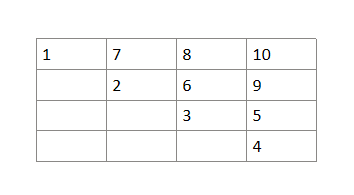Author: Abhishek Ghosh
Tester: Siddharth Garg
Editorialist: Chirag Saini, Saksham Negi

Easy

# PREREQUISITES

Math, Sequences and Series

# PROBLEM

You are given an integer N. In a grid of size N * N , numbers are filled starting from 1, diagonally from the top-left corner to the bottom-right corner. After reaching the end of the grid, you move upwards and repeat the same, in the other direction. This goes on till no more numbers can be filled in the grid. We need to calculate the sum of top and right border elements.

# QUICK EXPLANATION

• To understand the problem, we can divide the total sum of favorable elements in the grid into 3 algebraic series and find the general solution for them. The total sum (i.e the sum of these 3 algebraic series) would be a simple equation in terms of N (size of the grid) which is required to solve the question in O(1) time complexity.
• The formula that we finally derive is N(\frac{2N^2 + 1}{3})

# EXPLANATION

Subtask 1 can be passed by creating a grid and simulating the process.

Subtask 2 can be passed in O(N) time for each test case by simply running a loop, but this will TLE for larger values of N.

To pass subtask 3, we need to do a little better (O(1) solution for each test case)

The problem can be understood by considering the sum of all favorable elements that we get in the grid after all the numbers are filled to derive an O(1) mathematical formula .

\implies For example

Consider a 4 * 4 rock grid as follows :The final sum in this case is : 1 + 7 + 8 + 10 + 9 + 5 + 4
On Rearranging the elements we get : ( 1 + 5 + 8 + 10 ) + ( 4 + 7 + 9 + 10) - 10 (Corner Element)
We can consider these 3 algebraic series separately :
S_{1} : ( 1 + 5 + 8 + 10 + … + till N terms)
S_{2} : ( 4 + 7 + 9 + 10 + … + till N terms)
S_{3} : 10

Now Considering S1 :
1 + 5 + 8 + 10 + … N terms
WLOG (without loss of generality),
1 + (N+1) + 2N + … N Terms

Let us consider T_{n} to be an^2 + bn + c
So,
(n=1) T_{1} = a + b + c = 1
(n=2) T_{2} = 4a + 2b + c = N+1
(n=3) T_{3} = 9a + 3b + c = 2N

On solving these equations we get:
a = \frac{-1}{2}, b = N + \frac{3}{2}, c = -N

Therefore, for S_{1}, it’s general term T_{n} can be written as : T_{n} = \frac{-1}{2} n^2 + (\frac{N+3}{2})n + (-N)
We know that, S_{1} = \sum_{n=1}^{N} Tn

Hence we get :
\implies S_{1} = (\frac{-1}{2}) \sum n^2 + (N+\frac{3}{2}) \sum n - (N) \sum 1

Proof for S1

\implies S_{1} = \frac{-1}{2} ( (N)*(N+1)*(2*N+1) / 6) + (N+3/2)*((N)*(N+1) / 2) -N*(N)
\implies S_{1} = - ( (N^2+N) * (2*N+1) / 12) + ( (N^2+N)*(2*N+3) / 4 ) - N^2
\implies S_{1} = ((N^2 + N)*(4*N+8)/12) - N^2
\implies S_{1} = (N^2+N)*(N+2) / 3 - N^2 = (N^3+3*N^2+2*N-3*N^2)/3
\implies S_{1} = N*(N^2 + 2) / 3

\implies S_{1} = \frac{N*(N^2+2)}{3}

Now, Considering the corner element i.e S_{3} :
On carefully noticing, we come to the conclusion that S_{3} is nothing but the sum of first N natural numbers i.e \frac{N * (N+1)}{2}

Now Considering S_{2} :
4 + 7 + 9 + 10 + … N terms
WLOG,
N + 2N-1 + 3N - 3 + ...N terms

Let us consider T_{n} to be an^2 + bn + c
So,
(n=1) T_{1} = a + b + c = N
(n=2) T_{2} = 4a + 2b + c = 2N - 1
(n=3) T_{3} = 9a + 3b + c = 3N - 3
On solving these equations we get:
a = \frac{-1}{2}, b = N + \frac{1}{2}, c = 0

Therefore, for S_{2}, it’s general term Tn can be written as : T_{n} = (\frac{-1}{2})N^2 +(N+\frac{1}{2})N + 0
We know that, S_{2} = \sum_{n=1}^{N} Tn
Hence We get :

\implies S_{2} = \frac{-1}{2} \sum n^2 + (N+1/2) \sum n

Proof

\implies S_{2} = \frac{-1}{2} ( (N)*(N+1)*(2*N+1) / 6) + (N+1/2)*((N)*(N+1) / 2)
\implies S_{2} = - ( (N^2+N) * (2*N+1) / 12) + ( (N^2+N)*(2*N+1) / 4 )
\implies S_{2} = ((N^2 + N)*(4*N+2)/12)

\implies S_{2} = N(N+1)(2N+1) / 6

The Final Sum :
(S) = S1 + S2 - S3

Proof

(S) = N(\frac{N^2+2}{3}) + N(\frac{(N+1)(2N + 1)}{6}) - \frac{N(N+1)}{2}
(S) = \frac{N}{6}( 2N^2 + 4 + 2N^2 + 3N + 1 -3N - 3)
(S) = \frac{N}{6}(4N^2 + 2)

(S) = N\frac{(2N^2 + 1)}{3}

Therefore We get an O(1) solution which only depends on the size N of the grid .

# Solution

Setter's Solution
#include <bits/stdc++.h>
using namespace std;
typedef long long ll;

int32_t main() {
#ifndef ONLINE_JUDGE
freopen("input.txt", "r", stdin);
freopen("output.txt", "w", stdout);
#endif
ios_base::sync_with_stdio(false);
cin.tie(NULL);
cout.tie(NULL);

int T = 1;
cin >> T;

while(T--) {
ll n; cin >> n;
ll corner = (n*(n+1)) / 2;
ll s1 = (n * (n*n + 2)) / 3;
ll s2 = (n * (n+1) * (2*n+1)) / 6;
ll ans = s1 + s2 - corner;
cout << ans << "\n";
}

return 0;
}